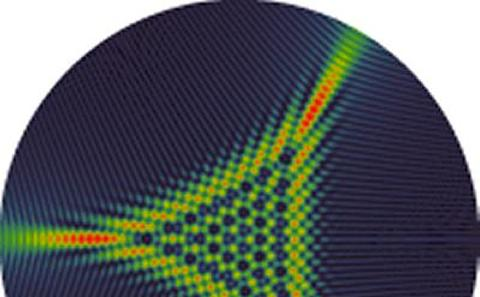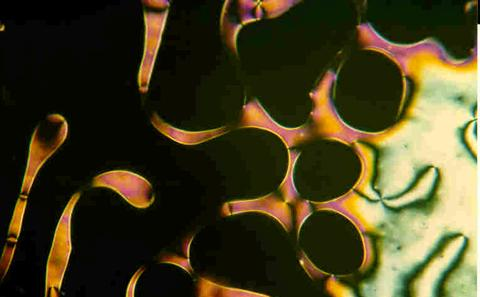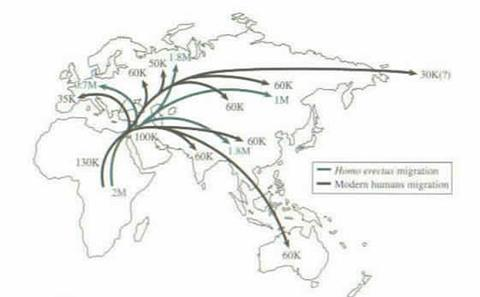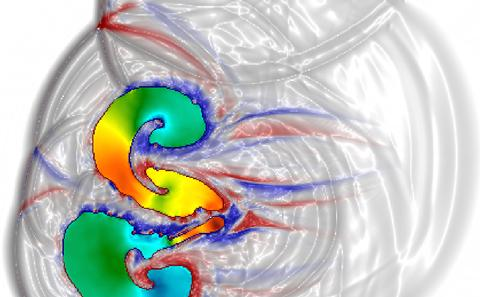The University of Southampton
Mathematical Sciences

# Research Group: Computational Applied Mathematics

Currently Active:
Yes

Mathematical modelling of biological, engineering and physical systems requires combining careful mathematical analysis and asymptotic expansions to powerful computational methods. We develop new asymptotic and computational methods and we apply them to a range of systems, spanning in size from molecules (liquid crystal, photovoltaic cells) to human beings (population modelling) and astrophysical objects (relativistic dynamics of neutron stars and black holes).## Asymptotic methods

Our studies in this field focus on two areas. The first is exponential asymptotics, i.e. how exponentially small terms that are usually neglected, may become dominant and determine the mathematical and physical behaviour of the system under study. The second is multiscale methods: the aim of these methods is to obtain equations for the macroscopic properties of a material or device, that incorporate in a rigorous way, the properties of if microstructure.## Computational multiscale modelling

We model materials and devices, such as liquid crystals, solar cells and metamaterials, which have structures on different length scales. For example, an electric battery can be described on three scales: atomic (chemical reactions), mesoscopic (electrode structure) and macroscopic (global current flow). To model such systems, we develop and use powerful computational and mathematical tools, ranging from a range of PDE solvers to equation-free methods.## Computational human sciences

We apply mathematics to the study of the human condition. Some of our studies involve human biology. Examples include modelling bioelectricity and understanding the role of active drainage flows in preventing Alzheimer’s disease. Other studies take the point of view of anthropology and social science. Here, for example, we have modelled the expansions of human populations across the world, and examined models of cooperation and competition in order to understand better how humans (and other animals) can live in large societies. Some of our studies lie in between these limits, such as when we study the genetics of selection, or the interaction of genetics and economics in the evolution of domestic animals. Finally, we are interested in the contemporary human condition, specifically how and whether we can avoid collapse by raw material shortage or global warming.## Computational physics

We model a variety of physical systems: superfluids in geometry that range in size from Bose Einstein condensates of cold atoms to neutron stars; combustion in flames and supernova explosions; relativistic shockwaves in astrophysical plasmas; collisions of black holes. Our research tackles both the mathematical foundations required for stable and accurate calculations, and the implementation of advanced finite difference and finite volume simulations on machines from desktops to massively parallel HPC resources.

List of related projects to Computational Applied Mathematics
Related ProjectsStatus
Computational PhysicsActive
Computational Human SciencesActive
Computational Multiscale ModellingActive
Asymptotic MethodsActive